# Wave Walker DSP

## DSP Algorithms for RF Systems

DSP for Beginners: Simple Explanations for Complex Numbers! The second edition includes a new chapter on complex sinusoids.

Single Pole IIR Filter: Substitute for Moving Average Filter
August 10, 2022

#### Introduction

The single pole IIR filter is incredibly efficient and can be a great stand-in for a moving average filter! In this blog I describe how to derive the 3 dB bandwidth of the IIR filter to be equivalent to a moving average filter by designing the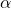weight. The design process builds on the IIR analysis from this DSP Stack Exchange question and my previous blog post.

More blog posts on DSP:

#### Single Pole IIR

The impulse response of the single pole IIR filter is

(1)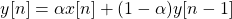which can also be written as

(2)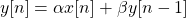where

(3)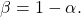The magnitude of the frequency response of a single pole IIR filter is given by

(4)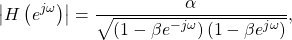where the magnitude-squared is

(5)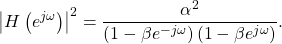#### Setting up the Design Problem

A previous blog post demonstrated how the 3 dB bandwidth of a moving average filter of length N can be approximated by

(6)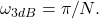The goal is to find the valuefrom (1) such that the 3 dB cutoff frequency of (5) is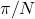.

#### IIR Filter Design Equation

The 3 dB cutoff corresponds to a magnitude-squared of 1/2. The design equation is therefore

(7)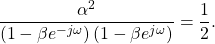Multiplying the complex exponential terms,

(8)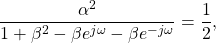which is simplified as

(9)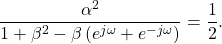(10)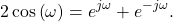Substituting (10) into (9),

(11)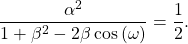Multiplying the terms of (11) results in

(12)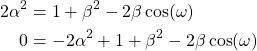Substituting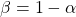,

(13)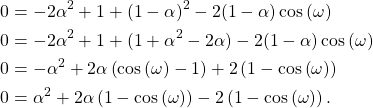#### Solving with the Quadratic Equation

The design equation (13) can be solved forusing the quadratic equation,

(14)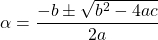where

(15)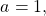(16)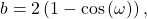(17)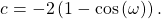Substituting (15), (16) and (17) into (14),

(18)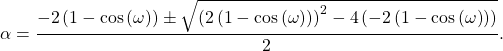Simplifying (18),

(19)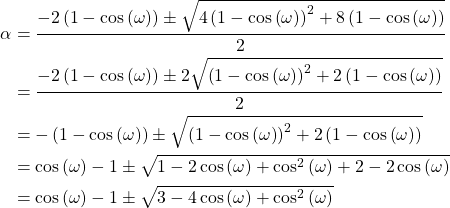#### Picking the Alpha Value

The design equation in (19) has two solutions due to the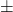term. Figure 1 shows the two solutions for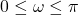.

The + from theis chosen from (19) to select the positive coefficient, therefore the final design equation is

(20)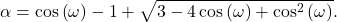To design a high pass filter (HPF), choose the – term in thefrom (19).

#### Approximating the Moving Average Filter

The 3 dB cutoff for a length N moving average filter from (6) is substituted into the design equation (20) and the magnitude of the frequency response is plotted in Figure 2 for multiple values of N.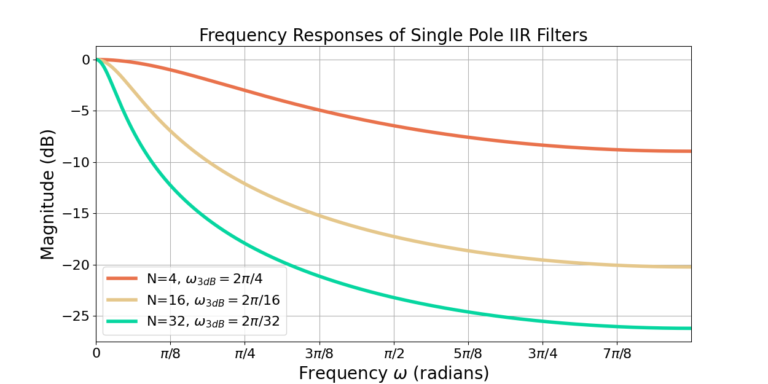Figure 2: The frequency response of single pole IIR filters with different cutoff frequencies. The magnitudes are normalized to a maximum gain of 0 dB.

#### Comparison to Moving Average Filter

Figure 2 shows that increasing the filter cutoff frequency also reduces the sidelobe attenuation. Although the single pole IIR filter uses less computation than a moving average filter, it also has lower attenuation of out of band frequency content. Figure 3 shows that the single pole IIR filter approaches the response  of the moving average filter for N=8 but with about 4 dB worse attenuation.